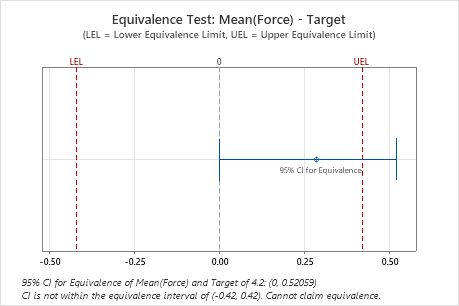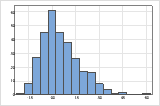# Interpret the key results for 1-Sample Equivalence Test

Complete the following steps to interpret a 1-sample equivalence test. Key output includes the estimate of the difference, the confidence interval, the equivalence plot, and other graphs.

## Step 1: Determine whether the population mean and the target are equivalent

Compare the confidence interval with the equivalence limits. If the confidence interval is completely within the equivalence limits, you can claim that the population mean is equivalent to the target. If part of the confidence interval is outside the equivalence limits, you cannot claim equivalence.Key Results: 95% CI, Equivalence interval In these results, the 95% confidence interval exceeds the upper equivalence limit. Therefore, you cannot claim that the population mean is equivalent to the target.
###### Note

If you selected an alternative hypothesis to test an inequality, rather than equivalence, evaluate the overall results by comparing the lower bound with the lower limit or the upper bound with the upper limit. For more information, go to Difference for 1-Sample Equivalence Test and click "Lower bound" or "Upper bound".

## Step 2: Check your data for problems

Problems with your data, such as skewness or outliers, can adversely affect your results. Use graphs to look for skewness (by examining the spread of the data) and to identify potential outliers.

### Determine whether the data appear to be skewed

When data are skewed, the majority of the data is toward the high or low side of the graph. Often, skewness is easiest to identify with a boxplot or histogram.For example, the right-skewed histogram shows salary data. Many employees are paid a relatively small amount, while increasingly few employees are paid large salaries. The left-skewed histogram shows failure rate data. A few items fail earlier while an increasing number of items fail later.

Data that are severely skewed can affect the validity of the test results if your sample is small (< 20 values). If your data are severely skewed and you have a small sample, consider increasing your sample size.

### Identify outliers

Outliers, which are data points that are far away from most of the other data, can strongly affect your results. Outliers are easiest to identify on a boxplot.